# Velocipedes

In the 19th century, bicycles did not have a chain drive, and the wheel axis connected the pedals directly. This wheel diameter gradually increased until the so-called high bikes (velocipedes) with a front-wheel diameter of up to 1.5 meters, while the rear wheel was only 45 cm. In 1891, Frederick Osmond reached 38 km/h on a bicycle, a world record. How many times a minute did the front wheel turn?

n =  134.4

### Step-by-step explanation: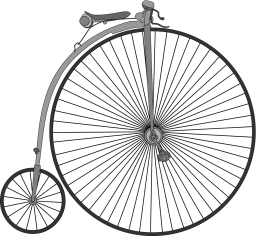Did you find an error or inaccuracy? Feel free to write us. Thank you!Tips to related online calculators
Do you want to convert length units?
Do you want to convert velocity (speed) units?
Do you want to round the number?
Do you want to convert time units like minutes to seconds?

#### You need to know the following knowledge to solve this word math problem:

We encourage you to watch this tutorial video on this math problem:

## Related math problems and questions:

• GearsThe front gear on the bike has 32 teeth and the rear, on the wheel, has 12 teeth. How many times does the bike's rear-wheel turn if you turn the right pedal 30 times? What distance will you go if the circumference of the bicycle wheel is 250 cm?
• Bicycle wheelA bicycle wheel has a diameter of 60 cm. Approximately how many times does the wheel rotate at 2.5 km long trip?
• A bicycle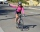A bicycle wheel has a diameter of 63 cm. Calculate how many times the wheel turns round in travelling 19.8 km .
• Bicycle gears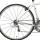The toothed wheel on the bicycle pedal has 40 teeth, the wheel on the rear wheel has only 16 teeth. How many times does the rear wheel turn if the pedals rotate 50 times?
• Bicycle wheel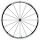Bicycle wheel diameter is 62 cm. How many times turns the bicycle on the road 1 km long?
• Wheel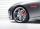How many times turns the wheel of a passenger car in one second if car run at speed 100 km/h. Wheel diameter is d = 62 cm.
• Cyclist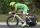A cyclist is moving at 35 km/h and follows a pedestrian who is walking at speed 4 km/h. Walker has an advantage 19 km. How long take a cyclist to catch up with him?
• Velocipede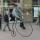The front wheel of velocipede from the year 1880 had a diameter 1.8 m. Suppose the front wheel turned again one, then the rear wheel 6 times. What was the diameter of the rear wheel?
• Saw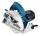Blade circular saw with a diameter 42 cm turns 825 times per minute. Expresses his cutting speed in meters per minute.
• Diameter of a cylinderThe diameter of the cylinder is 42 cm. How many times does the cylinder turn on a 66m long track?
• Wheel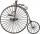Diameter of motocycle wheel is 52 cm. How many times rotates wheel on roand long 2 km?
• Runner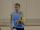Peter ran a speed of 12.4 km / h. After 45 minutes running he had a hiatus. The track is 31 km long. How many kilometers he still to run if he still running at the same speed?
• Average speed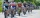The average speed of a pedestrian who walked 10 km was 5km/h, the average speed of a cyclist on the same track was 20km/h. In how many minutes did the route take more than a cyclist? Q
• Peleton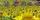The cycling race was run at an average speed of 45 km/h. One cyclist lost with defect 8 minutes. How long and how far he must go at speed 51 km/h to catch the peloton again?
• Circular arcCalculate the length of the circular arc if the diameter d = 20cm and the angle alpha = 142 °
• Wheel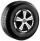What is the wheel diameter if on the 0.57 km track turns 106 times?
• BaseBase of building is circle with diameter 25 m. Calculate the circumference of a circular trench witch diameter is 41 cm wider than the diameter of the base.